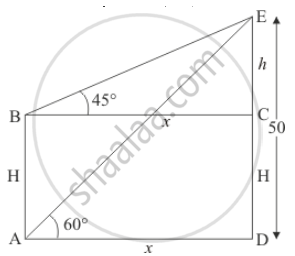# From the Top of a 50 M High Tower, the Angles of Depression of the Top and Bottom of a Pole Are Observed to Be 45° and 60° Respectively. Find the Height of the Pole. - Mathematics

From the top of a 50 m high tower, the angles of depression of the top and bottom of a pole are observed to be 45° and 60° respectively. Find the height of the pole.

#### Solution

Let H be the height of the pole, makes an angle of depression from the top of the tower to top and bottom of\ poles are 45° and 60° respectively.

Let AB = H , CE = hAD = x and DE = 50m.

∠CBE = 45^@ and ∠DAE = 60^@

Here we have to find height of pole.

The corresponding figure is as follows=> tan A = (DE)/(AD)

=> tan 60^@ = 50/x

=> x = 50/sqrt3

Again in ΔBCE

=> tan B = (CE)/(BC)

=> tan 45^@ = h/x

=> 1 = h/x

=> h = 50/sqrt3

=> h = 28.87

Therefore H = 50 - h

=> H = 50 - 28.87

=> H = 21.13

Hence height pole is 21.13 m

Concept: Heights and Distances
Is there an error in this question or solution?

#### APPEARS IN

RD Sharma Class 10 Maths
Chapter 12 Trigonometry
Exercise 12.1 | Q 46 | Page 32